## Paper on Dynamical Quantum Phase Transitions Published in PRL

Ever since their discovery in 2013, Dynamical Quantum Phase Transitions (DQPTs) have attracted great theoretical and experimental attention as quantum many-phenomena occurring at short time-scales. DQPTs are non-analytic points (sharp changes) occurring in the time-evolution of the fidelity density $$f$$, a quantity that indicates how far a state has evolved away from its initial condition and is zero if the system is exactly in its initial state.However, the mere observation of a DQPT, usually reliant on numerical calculations, does not shed light on the physical mechanisms that drive it. To address this open question, in our recent work Entanglement view of dynamical quantum phase transitions [Phys. Rev. Lett. 126, 040602 (2021)] we used a novel tensor-network based approach. Our work revealed the existence of two distinct physical mechanisms capable of driving DQPTs: if single-spin physics dominates the dynamics, one has precession-driven DQPTs (pDQPTs), whereas when spin-spin interactions dominate one has entanglement-driven DQPTs (eDQPTs).

To uncover these mechanisms, we made use of the efficient encoding of the quantum state afforded by infinite Matrix Product States (iMPS). The iMPS can be seen as a linear superposition of all possible product states generated by an “automaton”, as shown below: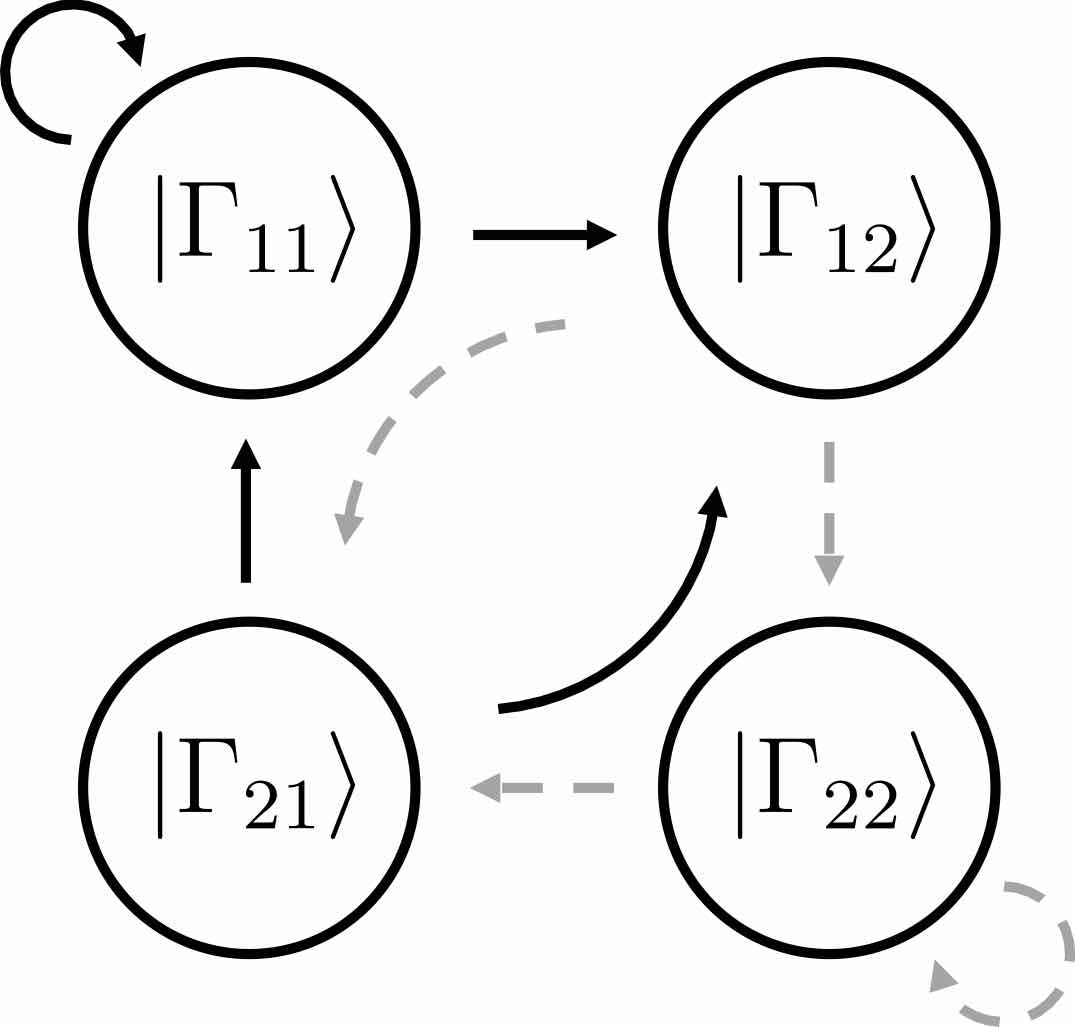Each circle in the automaton corresponds carries the local state $$|\Gamma_{ij}\rangle$$ at a site. The arrows give the allowed choices for the states at the following site, with weights given by square roots of the entanglement spectrum $$\lambda_i$$. The number of options available from each site, 2 in the above example, is known as bond dimension $$\chi$$. In our work, we showed that suitable $$\chi=2$$ Ansätze are able to capture the mechanisms of p- and eDQPTs. The fidelity density can be obtained by contracting the iMPS with the initial state, yielding the fidelity transfer matrix Tf. DQPTs are then caused by the second-largest eigenvalue of Tf, $$e_2$$, overtaking $$e_1$$ in magnitude, and this can happen for different reasons.

When the entanglement gap is large, $$\lambda_1 >>\lambda_2$$, the automaton prescribes that the state is approximately given by a product state $$|\Gamma_{11}\rangle$$, with corrections $$|\Gamma_{21,12}\rangle$$ at each site being weighted by $$\sqrt{\lambda_2/\lambda_1}$$. For example, for a system initialized in the all-down state, $$|\psi_1\rangle=|\downarrow\rangle$$ and $$|\psi_2\rangle=|\uparrow\rangle$$. The DQPT is then caused by an abrupt switch in the relative contribution of $$|\uparrow\rangle$$ and $$|\downarrow\rangle$$ as they precess respectively away from and towards the initial state. This is the physics of pDQPTs, and can be conveniently visualized on the Bloch sphere: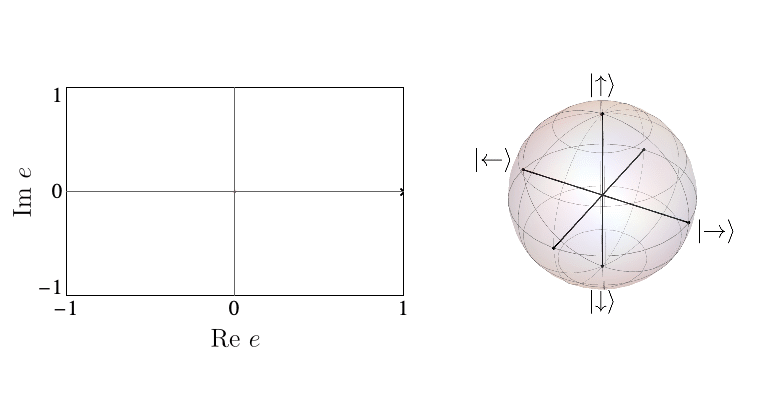In contrast, eDQPTs are driven by an avoided crossing in the entanglement spectrum. In this case, near the DQPT the quantum state undergoes a rearrangement whereby the initially off-diagonal component, which for $$\lambda_2 \ll \lambda_1$$ provides a correction to the leading top-diagonal component, becomes the dominant contribution. The automaton representation then shows that excitations over the dominant product state can be created at decreasing cost as the gap is reduced. After the avoided crossing the formerly subleading component will become leading; this corresponds to the swap of $$e_1$$, $$e_2$$ and signals an eDQPT:In our work, we showed how the mechanism driving a given DQPT is also reflected in the behaviour of a number of physical observables, including the mutual information and one-point functions, opening the door to the experimental probing of the physics behind DQPTs.

## Paper on mixed phase space appears at PRX

Intuition suggests that generic, strongly interacting quantum systems establish thermal equilibrium even in isolation. In the process of thermalization, quantum information encoded in the system becomes irrecoverable. Hence, it is highly desirable to understand how thermal equilibrium is established and if it can be delayed. In our recent paper, Phys. Rev. X, 10, 011055 (2020), we provide a framework that allows one to find the slowly thermalizing states in strongly interacting quantum systems.

Using mathematical techniques to map the quantum dynamics of a strongly interacting system into a classical nonlinear dynamical system, we show that the resulting dynamical system generally has a mixed phase space where regions with regular motion coexist with chaotic islands. This can be illustrated by using Poincare maps. The animation below shows an example of the periodic trajectory in the dynamical system obtained from a quantum model.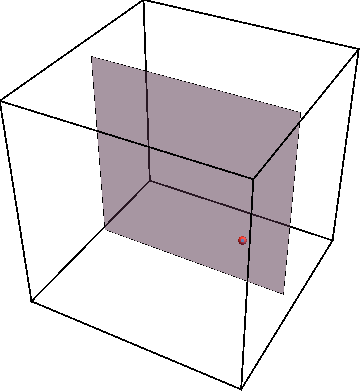If we now initialize system not on the periodic trajectory but close-by in parameter space, the new trajectory will remain close to the periodic one as the animation below shows.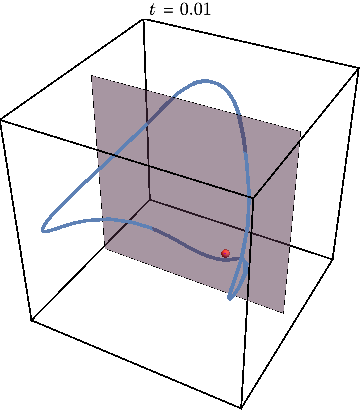Now we can get rid of third dimension by tracking the times when trajectory crosses the gray plane. The periodic trajectory reduces to a simple dot on such a Poincare map, while the nearby trajectory generates a circle  —  a cross-section of KAM torus:Finally, we may illustrate what happens when system is initialized far away from this periodic trajectory, resulting in chaos: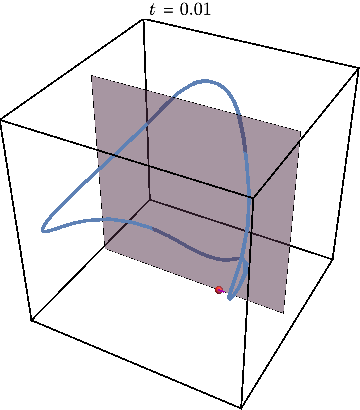The Poincare section now looks very different from before:Plotting the Poincare map for many different initial conditions shown by a different color, we obtain the figures used in the paper to illustrate the mixed character of phase space, where star shows location of periodic trajectory: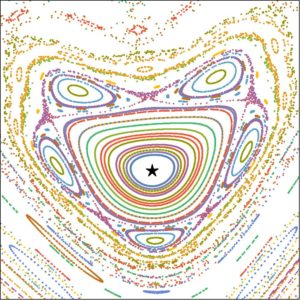Since the trajectory of interest are stable, i.e. cannot be described by Lyapunov exponent, we characterize the error encountered in approximating quantum dynamics by a classical dynamical system. When the error is small, initializing the quantum system in the regular region of phase space can give rise to spectacular many-body revivals, in which the system periodically returns to its original state. This generalizes the mechanism of quantum many-body scars—revivals that happen when there is an unstable periodic trajectory. However, even when the error is large, we show that our framework can find states with parametrically slower thermalization.

Our work provides a practical tool to search for slowly thermalizing states of strongly interacting quantum systems. Moreover, our work puts the intriguing connection between classical and quantum chaos in many-body systems on a firm basis.

## Mixed phase space in quantum many-body systems

In the earlier work we identified the quantum scars as a mechanism of weak ergodicity breaking in interacting many-body systems. The name “quantum many-body scars” was inspired by the presence of unstable TDVP trajectory reported in Ref. [Phys. Rev. Lett. 122, 040603 (2019)], thus allowing to make parallels with quantum scars in single-particle billiards.

However, in a few-body quantum systems quantum scarring is not the only possible mechanism of dependence of relaxation on initial state. In fact, the mixed phase space in a few body systems presents another common mechanism. In the most recent work arXiv:1905.08564 we generalized the concept of mixed phase space to strongly interacting quantum systems.

## Colloquium: Many-body localization, thermalization, and entanglement

Quantum systems of many particles, which satisfy the ergodicity hypothesis, are conventionally described by statistical mechanics. However, not all quantum systems are ergodic, with many-body localization providing a generic mechanism of ergodicity breaking by disorder. Many-body localized (MBL) systems remain perfect insulators at non-zero temperature, which do not thermalize and therefore cannot be described using statistical mechanics. In the Colloquium: Many-body localization, thermalization, and entanglement written together with D. Abanin, E. Altman, and I. Bloch and recently published at Reviews of Modern Physics, we summarize recent theoretical and experimental advances in studies of MBL systems, focusing on the new perspective provided by entanglement and non-equilibrium experimental probes such as quantum quenches.

## Paper on analytically solvable RG appears at PRL

Recent work on the analytically solvable RG for MBL transition by Anna Goremykina in collaboration with Romain Vasseur & Maksym Serbyn appears at PRL, see more details here: Analytically solvable renormalization group for the many-body localization transition. In short, in this work we suggest that MBL transition may be described by the Kosterlitz-Thouless universality class. More support for this scenario is available in the follow-up work, arXiv:1811.03103.

## Weak ergodicity breaking from quantum many-body scars

Quantum scars present an example of weak ergodicity breaking in a context of quantum chaos. In our recent work in Nature Physics, we also generalized this concept to the many-body case.

#### Quantum scars in a billiard

Imagine a ball bouncing around in an oval stadium. It will bounce around chaotically, back and forth through the available space. As its motion is random, it will sooner or later visit every place in the stadium as is illustrated in the example below:Amidst all the chaos, however, there might be a potential for order: if the ball happens to hit the wall at a special spot and at the “correct” angle of incidence, it might end up in a periodic orbit, visiting the same places in the stadium over and over and not visiting the others. Such a periodic orbit is extremely unstable as the slightest perturbation will divert the ball off its track and back into chaotic pondering around the stadium:The same idea is applicable to quantum systems, except that instead of a ball bouncing around, we are looking at a wave, and instead of a trajectory, we are observing a probability function. Classical periodic orbits can cause a quantum wave to be concentrated in its vicinity, causing a “scar”-like feature in a probability that would otherwise be uniform. Such imprints of classical orbits on the probability function have been named “quantum scars”. Below we compare the “scarred“ eigenstate in the stadium with the more typical state: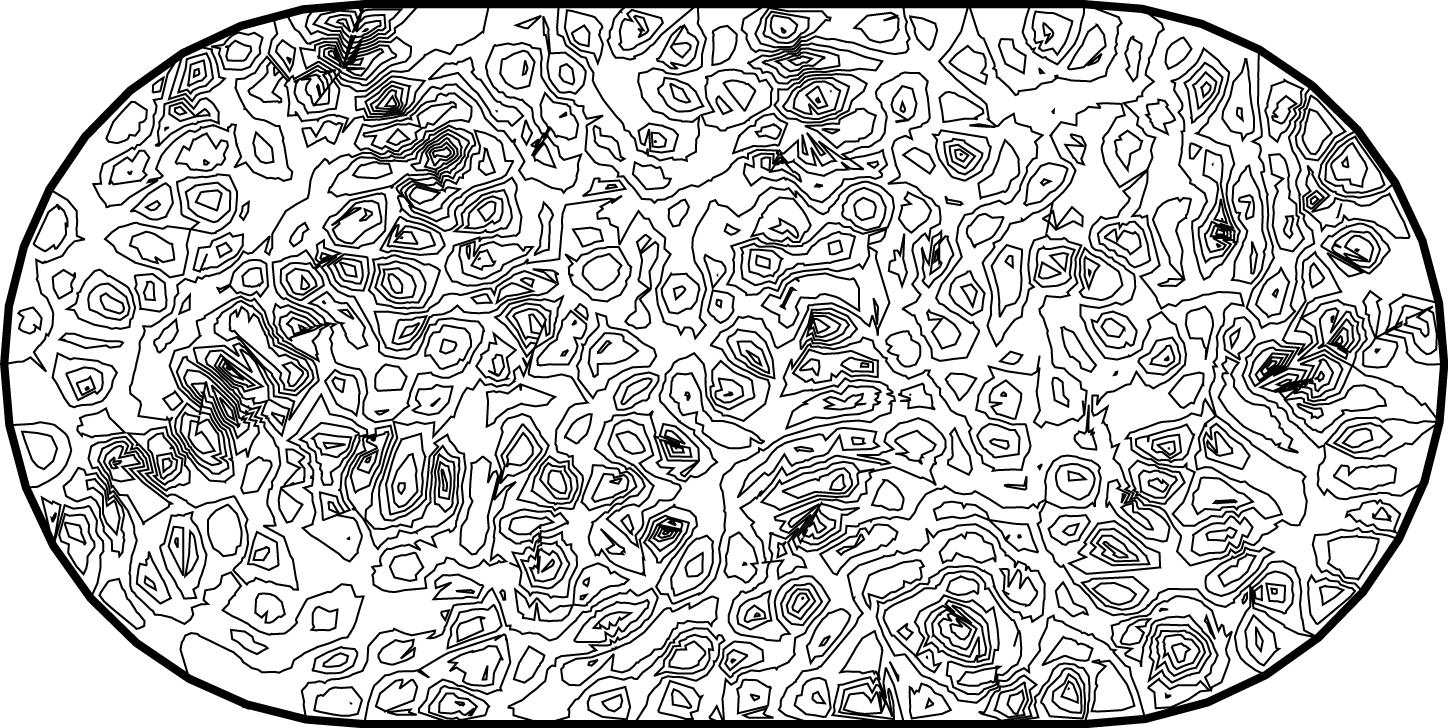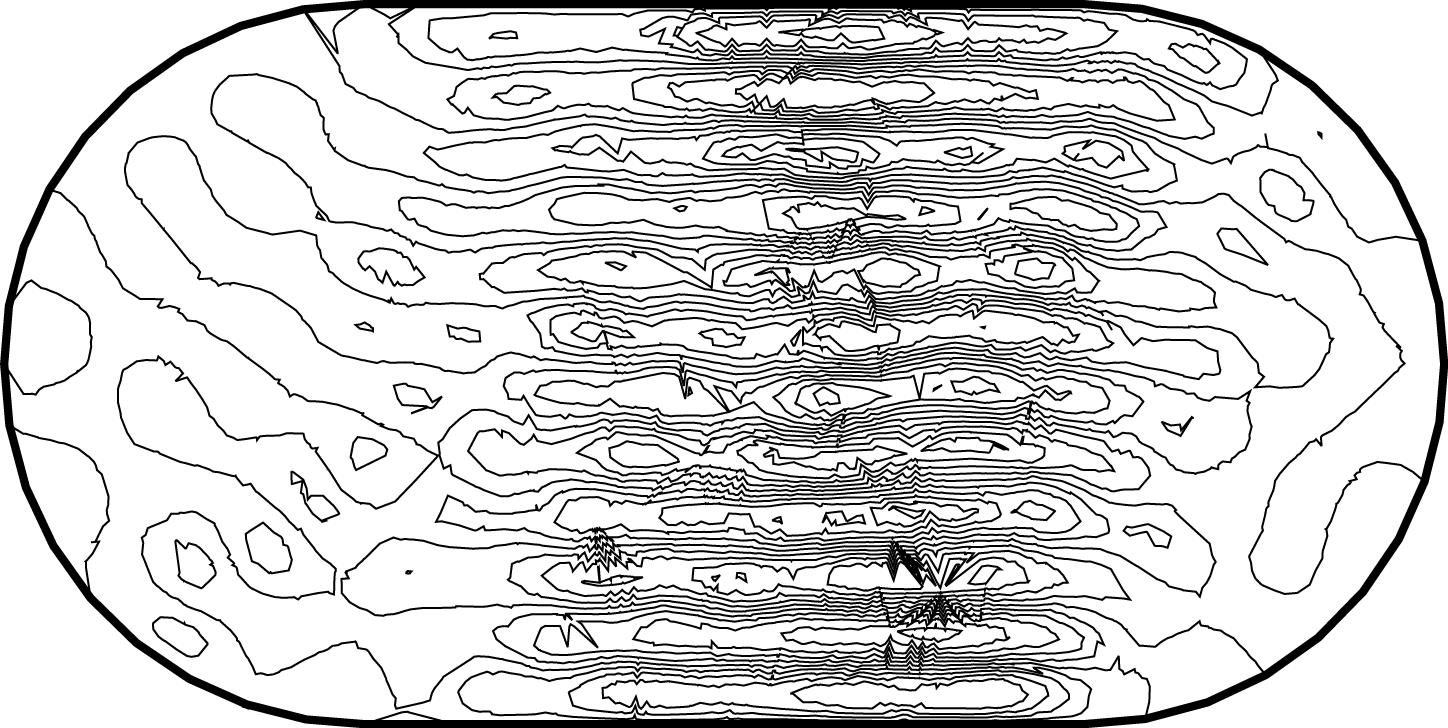#### Quantum many-body scars

We observed quantum-scarred eigenstates in the theoretical model that describes a chain of Rydberg atoms. All atoms in the chain can be in two possible states: excited and ground state. Moreover there exist a constraint that prohibits two excited atoms to be adjacent to each other. We found a coherent oscillations in such a system which underlie the quantum-scarred eigenstates. Below we show the animated cartoon of this trajectory for L=8 atoms.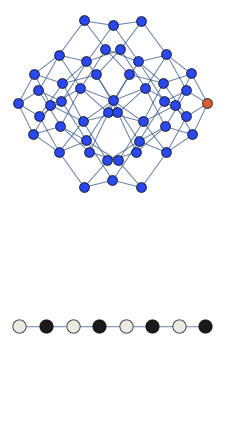Here the graph shows the space of all possible configurations, and the bottom shows the average density of excitation on each site. Animation shows oscillations between two patterns.

## Probing dynamics by matrix elements

In order to determine generic non-equilibrium dynamics of the generic local observable O one needs two ingredients. The spectrum of the many-body Hamiltonian and the matrix elements of the operator O in the basis of eigenstates completely specify evolution of the local observable O. Moreover, the matrix elements at small energy differences correspond to the long-time dynamics that may be very hard to access in the thermalizing system.

Using these insights, we studied the properties of the matrix elements across the many-body localization transition in the recent preprint arXiv:1610.02389. In particular, we used matrix elements to define the (many-body) Thouless energy ETh which corresponds to the inverse time scale of relaxation. The behavior of this Thouless energy reveals the critical fan preceding localization transition. In addition, we associate the critical region with the onset of broad distributions and typicality breakdown.